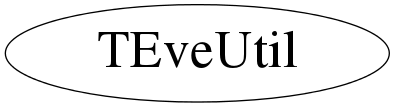# class TEveUtil

```
Standard utility functions for Eve.
```

## Function Members (Methods)

public:
 TEveUtil() TEveUtil(const TEveUtil&) virtual ~TEveUtil() static void AssertMacro(const char* mac) static Bool_t CheckMacro(const char* mac) static TClass* Class() static void ColorFromIdx(Color_t ci, UChar_t* col, Bool_t alpha = kTRUE) static void ColorFromIdx(Color_t ci, UChar_t* col, Char_t transparency) static void ColorFromIdx(Float_t f1, Color_t c1, Float_t f2, Color_t c2, UChar_t* col, Bool_t alpha = kTRUE) static Color_t* FindColorVar(TObject* obj, const char* varname) static Float_t GetFraction(Float_t minM, Float_t maxM, Float_t minQ, Float_t maxQ) virtual TClass* IsA() const static Bool_t IsU1IntervalContainedByMeanDelta(Float_t meanM, Float_t deltaM, Float_t meanQ, Float_t deltaQ) static Bool_t IsU1IntervalContainedByMinMax(Float_t minM, Float_t maxM, Float_t minQ, Float_t maxQ) static Bool_t IsU1IntervalOverlappingByMeanDelta(Float_t meanM, Float_t deltaM, Float_t meanQ, Float_t deltaQ) static Bool_t IsU1IntervalOverlappingByMinMax(Float_t minM, Float_t maxM, Float_t minQ, Float_t maxQ) static void LoadMacro(const char* mac) static void Macro(const char* mac) TEveUtil& operator=(const TEveUtil&) static void SetColorBrightness(Float_t value, Bool_t full_redraw = kFALSE) static void SetupEnvironment() static void SetupGUI() virtual void ShowMembers(TMemberInspector&) virtual void Streamer(TBuffer&) void StreamerNVirtual(TBuffer& ClassDef_StreamerNVirtual_b)

## Data Members

private:
 static TObjArray* fgDefaultColors

## Class Charts## Function documentation

void SetupEnvironment()
``` Setup Include and Macro paths.
Since inclusion into ROOT this does nothing but could
potentially be reactivated if some common macros are established
and shipped with binary ROOT (in macros/eve). For example, these
might be used to spawn specific GUI / GL configurations.
```
void SetupGUI()
``` Setup icon pictures and mime-types.
```
Bool_t CheckMacro(const char* mac)
``` Checks if macro 'mac' is loaded.
```
void AssertMacro(const char* mac)
``` Load and execute macro 'mac' if it has not been loaded yet.
```
void Macro(const char* mac)
``` Execute macro 'mac'. Do not reload the macro.
```
``` Makes sure that macro 'mac' is loaded, but do not reload it.
```
void ColorFromIdx(Color_t ci, UChar_t* col, Bool_t alpha = kTRUE)
``` Fill col with RGBA values corresponding to index ci. If alpha
is true, set alpha component of col to 255.
ROOT's indexed color palette does not support transparency.
```
void ColorFromIdx(Color_t ci, UChar_t* col, Char_t transparency)
``` Fill col with RGBA values corresponding to index ci and transparency.
ROOT's indexed color palette does not support transparency.
```
void ColorFromIdx(Float_t f1, Color_t c1, Float_t f2, Color_t c2, UChar_t* col, Bool_t alpha = kTRUE)
``` Fill col with weighted RGBA values corresponding to
color-indices c1 and c2. If alpha is true, set alpha component
of col to 255.
```
Color_t* FindColorVar(TObject* obj, const char* varname)
``` Find address of Color_t data-member with name varname in object
obj.

This is used to access color information for TGListTreeItem
coloration from visualization macros that wrap TObjects into
TEveElementObjectPtr instances.
```
void SetColorBrightness(Float_t value, Bool_t full_redraw = kFALSE)
``` Tweak all ROOT colors to become brighter (if value > 0) or
darker (value < 0). Reasonable values for the value argument are
from -2.5 to 2.5 (error will be printed otherwise).
If value is zero, the original colors are restored.

You should call TEveManager::FullRedraw3D() afterwards or set
the argument full_redraw to true (default is false).
```
Bool_t IsU1IntervalContainedByMinMax(Float_t minM, Float_t maxM, Float_t minQ, Float_t maxQ)
``` Return true if interval Q is contained within interval M for U1 variables.
It is assumed that all values are within the [-2pi, 2pi] interval and
minM <= maxM & minQ <= maxQ.
```
Bool_t IsU1IntervalOverlappingByMinMax(Float_t minM, Float_t maxM, Float_t minQ, Float_t maxQ)
``` Return true if interval Q is overlapping within interval M for U1 variables.
It is assumed that all values are within the [-2pi, 2pi] interval and
minM <= maxM & minQ <= maxQ.
```
Float_t GetFraction(Float_t minM, Float_t maxM, Float_t minQ, Float_t maxQ)
``` Get fraction of interval [minQ, maxQ] in [minM, maxM]
```
Bool_t IsU1IntervalContainedByMeanDelta(Float_t meanM, Float_t deltaM, Float_t meanQ, Float_t deltaQ)
Bool_t IsU1IntervalOverlappingByMeanDelta(Float_t meanM, Float_t deltaM, Float_t meanQ, Float_t deltaQ)
virtual ~TEveUtil()
`{}`